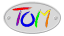# The Worlds Shortest C Implementation of Rot13

```main(a){while(a=~getchar())putchar(~a-1/(~(a|32)/13*2-11)*13);}
```
written by Michael Schroeder (mlschroe@faui43.informatik.uni-erlangen.de).
64 characters, including line feed.

## What's Rot13?

Rot13 is a simple "encryption" algorithm designed to make text illegible, but very easily "decrypted". It's used on USENET to post material that may be offensive - the reader has to choose to convert it back to plain text. Rot13 simply adds 13 to the value of each character, and wraps around back to "A" when it gets to "Z". So "A" becomes "N", "B" becomes "O", and "N" becomes "A". It works on both upper and lower case characters and leaves non-alphabetic characters as they were.

## History

A friend, J Greely, made a passing comment once that he had seen a very small C implementation of rot13, on the order of 120 characters, so I smugly replied that I could easily fit rot13 on one (standard terminal) line.

I was going to go after a mathematical implementation that probably would've ended up like the one shown above, except that I just couldn't figure it out. So I switched to a boolean algebra solution. Here's the derivations I went through to get an implementation of 81 characters, including the line feed:

```main(){int a,b;while((a=getchar())!=-1)putchar(a&64?(b=a&159)&&b<27?((b+12)%26+1)|(a&224):a:a);}

main(){int a,b;while((a=getchar())!=-1)putchar(a&64&&(b=a&159)&&b<27?((b+12)%26+1)|(a&96):a);}

main(){int a,b;while((a=getchar())!=-1)putchar((b=64^a&223)&&b<27?((b+12)%26+1)|(a&96):a);}

main(){int a,b;while((a=getchar())>=0)putchar((b=64^a&223)&&b<27?a&96|(b+12)%26+1:a);}

main(a,b){while((a=getchar())>=0)putchar((b=64^a&223)&&b<27?a&96|(b+12)%26+1:a);}

main(a,b){while((a=getchar())+1)putchar((b=64^a&223)&&b<27?a&96|(b+12)%26+1:a);}

main(a,b){while((a=getchar())+1)putchar((b=64^a&95)&&b<27?a&224|(b+12)%26+1:a);}
```
The second to last won me the "Best One-Liner" category in the 1991 International Obfuscated C Code Contest . The last one is similar but simoultaneously strips the seventh bit (the winner worked on characters regardless of whether or not the eighth bit was set.

Alas records are made to be broken. A discussion in alt.sources was brought to my attention. It seems someone came up with a lame ~200 character implementation. But by the time I had taken a look, there had been several other suggestions, including Michael Schroeder's above, and another that was only one character longer than mine, but used a remarkably straight-forward method:

```main(c){while((c=getchar())!=EOF)putchar(isalpha(c)?tolower(c)<'n'?c+13:c-13:c);}
```
This was written (or at least posted :-) by Duane Paulson (dap@netcom.com). I should note that this could easily be trimmed to shorter than mine by using the space-saving EOF check that I used:
```main(c){while((c=getchar())+1)putchar(isalpha(c)?tolower(c)<'n'?c+13:c-13:c);}
```

Perhaps we've finally seen the smallest possible implementation now. But somehow I doubt it.

4/15/98

O.K., someone brought an even shorter implementation (46 characters) to my attention. Here it is:

```main(){system("tr [a-zA-Z] [n-za-mN-ZA-M]");}
```
written by Jonathan H N Chin (jc254@newton.cam.ac.uk)

I'm not going to count it as the official version for three reasons. First, as the author points out, it isn't completely portable (mostly though). Second, it doesn't actually implement its own rot13 algorithm. Third, tr is itself actually written in C, and by its inclusion, kinda sorta makes the code much longer.Fine's HomeSend Me Email